Courses

# Test: Equilibrium of Rigid Bodies

## 10 Questions MCQ Test Physics Class 11 | Test: Equilibrium of Rigid Bodies

Description
This mock test of Test: Equilibrium of Rigid Bodies for JEE helps you for every JEE entrance exam. This contains 10 Multiple Choice Questions for JEE Test: Equilibrium of Rigid Bodies (mcq) to study with solutions a complete question bank. The solved questions answers in this Test: Equilibrium of Rigid Bodies quiz give you a good mix of easy questions and tough questions. JEE students definitely take this Test: Equilibrium of Rigid Bodies exercise for a better result in the exam. You can find other Test: Equilibrium of Rigid Bodies extra questions, long questions & short questions for JEE on EduRev as well by searching above.
QUESTION: 1

### Arrange the comparative stabilities of the three objects shown in the figure in terms of work and potential energy.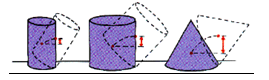Solution:

A object’s stability depends upon its centre of gravity.the larger the centre of gravity the more stable,By looking at the figures we can easily figure out which is more stable.

QUESTION: 2

### The figure shows a dish kept at an orientation with a marble placed on it. If the marble in figure is displaced slightly, it would rattle about for a while and come to rest in its original place. This is an example of :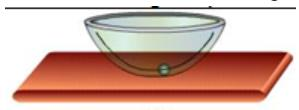Solution:

If a dish is kept at an orientation with a marble placed on it and if the marble in figure is displaced slightly, it would rattle about for a while and come to rest in its original place.  This is the example of Stable Equilibrium.
When a body tries to regain its equilibrium position after being slightly displaced and released, it is said to be in stable equilibrium.

QUESTION: 3

### The rock has a mass of 1 kg. What is the mass of the measuring stick if it is balanced by a support force at the one-quarter mark? The centre of mass of the measuring stick is at the one-half mark, one-quarter of the stick’s length beyond the pivot.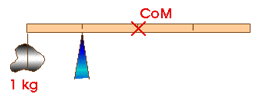Solution:

The stick is balanced by the 1 kg rock that is one-quarter of its length to the left and by its own weigh which is at the Centre of Mass, one-quarter of its length to the right.  Therefore, the mass of the stick must be one kilogram, 1 kg.

QUESTION: 4

A pair of equal and opposite forces with different line of action are said to form a

Solution:

When two forces of equal magnitude opposite in direction and acting along parallel straight lines, then they are said to form a couple. The perpendicular distance between the two force forming a couple is called the arm of the couple.

QUESTION: 5

A meter rule is supported at its centre. It is balanced by two weights, A and B. If A and B are placed at a distance 20 cm, and 40 cm from the centre of scale, find the weight of B. The weight of A is 50N.

Solution:

In balanced condition torque of the both sides must be equal so Fa × Da = Fb × Db
50 × 20 = Fb × 40
Fb = 25 N

QUESTION: 6

In order to rotate a body, we need to apply

Solution:

In order to rotate a body, we need to apply two equal forces at two ends but in the opposite direction.

QUESTION: 7

Determine the force that must be applied to end of the class 1 lever shown below to lift the 500 lb load.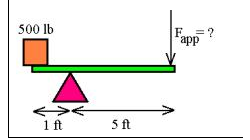Solution:

Balance the 2 opposite moments of inertia on the 2 sides
1 × 500 = F × 5
F = 100

QUESTION: 8

Five forces are separately applied to a flat object lying on a table of negligible friction as shown in figure. The force which will not cause the object to rotate about the centre of the object is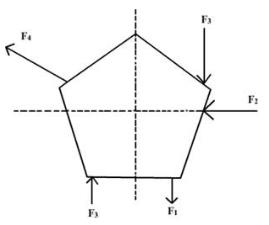Solution:

The line of action of F2 passes through the centre of rotation, and thus cannot produce a torque about the centre (r = 0).

QUESTION: 9

Which of the following appliance works on the principle of moments.

Solution:

In beam balance we consider the torque at centre of beam which is moment of force at its each end.

QUESTION: 10

There are two blocks of mass 3 kg and 5 kg hanging from the ends of a rod of negligible mass. The rod is marked in eight equal parts as shown. At which of the points indicated should a string be attached if a rod is to remain horizontal when suspended from the string?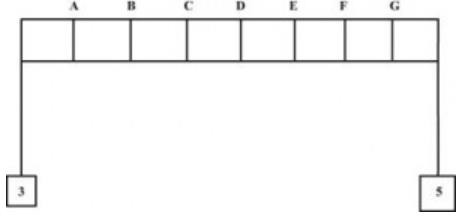Solution:

This is based on torque balance, That is F.d=const.
Therefore 3×d=5×(8-d)
by solving we get d=5.
and at point 5=E